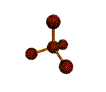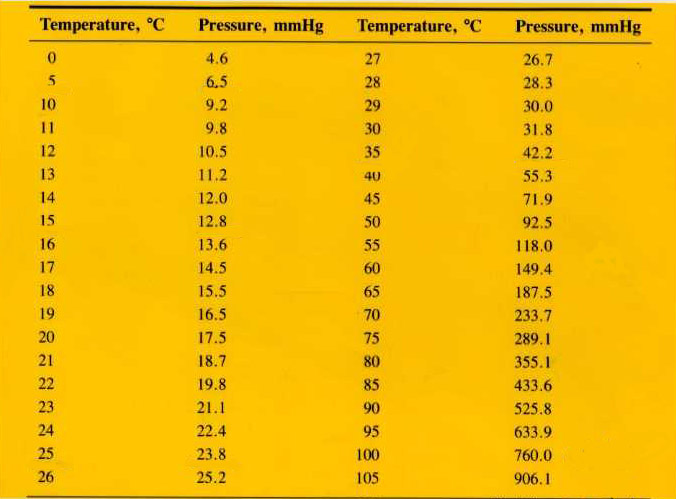##Mr. Rapp's Chemistry Help Site
 Grades  Graphing Tips  Online 3-D Laboratory  Reference Desk  AP Chemistry Test  News from Science  Links  After School Help  Fun Stuff  Teachers' Lounge    BHSS Chemistry  E-mail Mr. RappStudy Guide:  Chapter 13, Gas Laws Students should be able to... Explain the kinetic theory of matter as it applies to gases. Identify the properties of an ideal gas vs. a real gas Know units of pressure in atm, torr, mm Hg, and in Hg (HONORS: psi, kPa also) Calculate using Boyle's Law, Charles' Law, Gay-Lussac's Law, Combined Gas Law, and Ideal Gas Law (using 0.0821 for R). Change gases to STP. Calculate partial pressure of a gas using Dalton's Law. Calculate stoichiometric problems using density of a gas at STP. Find the molar volume of a gas (laboratory) Chemistry Gas Laws Review Work the following problems. 1. Given 500 cm3 of methane gas at 2.5 atm and 20 oC. What would be the volume of the gas at STP? 2. Given 6.0 liters of a gas at STP, what happens to the volume if the pressure is increased to 1432 mm Hg without changing the temperature? 3. A gas occupies 450 mL at 120 oC. If pressure does not change, to what Celsius temperature must the gas be heated for the volume to increase to 550 mL? 4. What pressure is exerted by 2 moles of a gas in a 500 cm3 container at 25 oC? 5. Two hundred cubic centimeters of a gas are collected by water displacement. The conditions at time are 1.1 atm and 30 oC. The vapor pressure of water at 30 oC is 23.4 mm Hg. What would be the pressure of the dry gas ? 6. What volume will 20.0 g of Argon occupy at STP? 7. How many moles of gas would be present in a gas trapped within a 100.0 mL vessel at 25.0 °C at a pressure of 2.50 atmospheres? 8. Find the mass (in grams) of 7.2 L of chlorine gas at 19°C and 28.71 in Hg.  What would the volume of this gas be at STP? 9.  What is the volume (in mL) of a gas at STP if the volume is 2.1 L at 40°C and 18.3 psi?  10.  Oxygen gas is produced by the decomposition of potassium chlorate by water displacement (over water).  What is the pressure of the “dry” oxygen gas if the pressure inside the gas measuring tube is 795 mm Hg and the temperature of the water is 28°C?11.  What is the pressure of oxygen in air if the pressure of nitrogen is 0.76 atm, and all the other gases (Ar, CO2, etc.) total 0.03 atm ? 12.  A sample of Ar gas is at 1.2 atm, 10°C, and has a volume of 11.1 L.  What is the temperature in °C if the gas is pressurized to 810 torr and now has a volume of 8.7L ? 13.  What is the pressure of dry hydrogen gas collected over water if the temperature is 23°C and the pressure is 842 torr? 14. 2 C2H6 + 7 O2 --> 4 CO2 + 6 H2O How many milliliters of ethane (C2H6) at STP are required to produce 22 g of carbon dioxide? 15. Using the reaction from problem 14, find how many liters of CO2 would be produced at STP if 96 g of ethane and an excess of oxygen were provided.E-mail Mr. Rapp | BHSS Chemistry Web SiteLast updated on August 29, 2011 8:14 AM